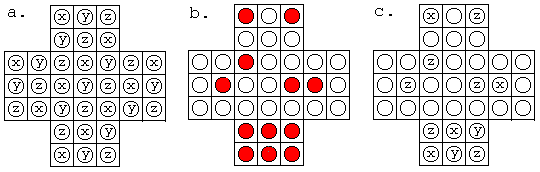top of page
All Posts: Blog2

# [Math Evenings] Peg Solitaire and Cyclic Groups

Among the diverse fields of mathematics, I love to see how seemingly distant concepts magically connect to produce beautiful proofs. Peg Solitaire and group theory is an example of such, as an elegant solution is used to prove a seemingly unprovable conundrum.

But before entering the talk on peg Solitaire, I wish to introduce some new concepts about groups. (These are not necessarily related to the solution for Peg Solitaire)

Definitions

Group Homomorphism

Given two groups (G,*) and (H,.), a group homomorphism from (G,*) to (H,.) is a function h such that

If such a homomorphism is bijective, we also call that to be a group isomorphism.

Cyclic Groups

Remember that we talked about infinite groups such as rational numbers-{0} under multiplication? But for a group to have finite cardinality, the elements must produce one another after applying the binary operator. In other words, the set must be "cyclic."

In a more rigorous way,

Order

In a cyclic group, k is the order of g when

and k is the smallest integer to satisfy this claim.

In the example of {1,3,5,7} and multiplication under modulo 8, the order of the element 1 would be 4.

Peg SolitairePeg Solitaire is a game played with 32 pegs and a cross-shaped board, in which the pegs can be moved around the 33 holes. The middle hole of the board is left empty, and the player can move a peg so that the peg "jumps over" the other peg. But the peg that had been jumped over should be removed from the board. (Gif in the right)

Then, anyone who leaves the last peg in the center is a genius, and anyone who leaves a single peg elsewhere is an outstanding player. But after playing this game by yourself, you might wonder: is it possible to have the final peg in all 33 holes? Or can the final peg be located in only some of the 33 holes?

The seemingly unrelated concept of group theory play a role in this question. Firstly, we will label all the 33 holes as Fig 1 a. If the pegs are left as in Fig 1 a, we can represent the configuration as Fig 1 c.Fig 1. placing letters onto the board

With this special notation, we will define an operation + for x, y, and z. If a peg moves from a hole denoted x over a hole y to a hole z, we will write it as

Then, note that

Hence, this binary operator is commutative. For the sake of having an inverse and identity element, we will define

This is especially true if you consider what + means. Jumping x over x would basically have no change, which is the identity element. Then, each of the elements now have an identity element. Also, associativity can be proven by trying the 27 possible combinations.

Note that from the definition of an identity,

Now, we can discover that there is an invariant for any configuration of any number of pegs. In the starting configuration, the sum of the symbols for all occupied positions is y. (This can be done by simple arithmetics) But when we have one peg on x move to a hole y, it would land on z. Do you see the connection with x+y+z being the identity? Regardless of what move we make, the sum of the occupied holes will be y.

Hence, the pegs can only be on the holes that say y on it. But this is not the end. Note that the board is line and rotationally symmetrical, so the points that are not line and rotationally symmetric must be excluded. For instance, in Fig 2 a, the asymmetric points would be able to obtain when the peg is in the current direction, but would be impossible to obtain when the peg is 90 degrees rotated. This is a contradiction, so we can get rid of the asymmetric points.FIg 2. Line and rotation symmetry

Therefore, the only possible places to end would be Fig 3.Acknowledgements

The graphics were found in: https://www.cut-the-knot.org/proofs/PegsAndGroups.shtml

22 views

See All
bottom of page